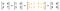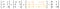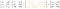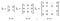# Introduction to Matrices

Matrices are a fundamental concept in AI, especially when working with neural networks and the majority of sub-fields of machine learning, such as image processing and synthesising, natural language processing, prediction — just about all types of deep learning models rely on matrices to contain and manipulate numerical collections of data.

Matrices are multi-dimensional arrays of numbers. They, and neural networks in general, are often referred to as “black boxes”, as they are notoriously hard to analyse for patterns or effectiveness.

## Matrices: Black boxes of multi-dimensional data

Matrices can be very large and can exist in a multitude of dimensions, typically ranging in the thousands if not millions of elements in medium to large-scale projects. Although they are very good at adjusting to network parameters while they find their way to a resulting output, it is rather challenging for us humans to understand what is manipulated within these vast collections of numbers, at each layer of the network. Indeed, model analysis and evaluation is a sub-field in and of itself within the larger scope of AI.

Think of an input of a neural network, perhaps a set of 1000 normalised features. These features would be passed into the network as a matrix of numbers, and manipulated at each layer of the network (by other matrices!) until the output layer is reached.

Neural networks, being functions that learn how to be efficient and output reasonable data, are black boxes in and of themselves — the programmer or engineer can give the network data to work with, but ultimately the complex formulation of matrix manipulations within the network define its effectiveness.

## What this piece will cover

Matrices are blocks of data (often floats) that can exist in 2 or more dimensions (think multi-dimensional arrays), the values of which are often in normalised or trained form. But how are they manipulated exactly? E.g. how would we add two matrices together — or multiply them?

The following sections will cover these basic operations that are common in deep learning. We will also cover more fundamental concepts with regards to matrices, including “special matrices” like the identity matrix and inverse matrices.

NumPy based examples of these matrix operations will also be provided to demonstrate how they are manipulated in real-world uses.

# Basic Matrix Operations

This section walks through the most commonly used matrix operations, and how they are performed with NumPy in Python.

## Addition

Adding two or more matrices together is the simplest of matrix operations, and just involves adding the corresponding numbers from each row and column. For addition:

• Each matrix needs to have the same dimensions.
• Each element of the resulting matrix is the sum of the corresponding elements of the matrices being added together.

Consider the following simple example where we add two 2 x 2 matrices together:Addition of two 2 x 2 matrices

This also holds true when dealing with negative and positive values together:Addition of two 3 x 2 matrices

In NumPy, adding matrices can be done either with NumPy’s `add` function, or simply with the `+` operator:

`""" Matrix Addition """import numpy as nparr1 = np.array([[7, 1], [-3, 7], [-2, -9]])arr2 = np.array([[-2, 6], [9, -7], [8, -1]])# addition with `np.add`arr3 = np.add(arr1, arr2)# addition with `+`arr3 = arr1 + arr2print(arr3)`

In both cases, the same matrix will result:

`print(arr3)>>>    [[  5   7]    [  6   0]    [  6 -10]]`

## Subtraction

Matrices are subtracted by subtracting each element of the corresponding row and column of two matrices. Just like addition, the matrices subject to the subtraction must be of the same dimensionality.

Here is an example:Subtracting two 3 x 2 matrices

Within Python, subtraction can be done with either NumPy’s `subtract` function or the `-` operator:

`""" Matrix Subtraction"""​import numpy as np​arr1 = np.array([[2, -3], [5, 9], [-4, -0]])arr2 = np.array([[7, 8], [14, -72], [-40, 95]])​# subtraction with `np.subtract`arr3 = np.subtract(arr1, arr2)​# subtraction with `-`arr3 = arr1 - arr2`

The result will yield the same result as the example above:

`print(arr3)>>>  [[ -5 -11]   [ -9  81]   [ 36 -95]]`

## When is matrix addition and subtraction useful?

Adding (or subtracting) matrices is very useful within neural networks when bias is introduced at a particular neuron. Bias introduces a slight manipulation over the input matrix at each neuron, and will entirely depend on your trained model. Bias is not manually configured, and is instead trained as your model is further optimised.

Matrix multiplication is slightly more complex than our simple operations so far. Let’s take a look at multiplication next, and how it is used in AI.

# Matrix Multiplication

Matrix multiplication follows a slightly more verbose methodology than simple addition or subtraction. When multiplying matrices, each row of the previous matrix multiplies with each column of the next matrix. This will become clearer in the example to follow.

One way to think of this process is that each row of the first matrix processes each column of the second matrix. Let’s firstly present two 2 x 2 matrices to multiply:

To calculate the final matrix (that is currently empty) each row of the first matrix needs to multiply with every column of the second matrix. The resulting matrix is known as the dot product of the matrices being multiplied.

Dot products are widely used in machine learning, with NumPy supplying their own `dot` function to leverage.

This process is admittedly is extremely hard to visualise, so let’s break it down into stages. The first row and column process resembles the following:

Notice that we’re starting with the first row of matrix 1, and the first column of matrix 2. The corresponding values are multiplied, with their products added together resulting in one dot product value.

Since there are more columns to multiply, row 1 then multiples with the next column of matrix 2:

Now row 1 of matrix 1 has processed all columns of matrix 2, we can repeat the whole process for row 2:

And finally process the last column of matrix 2 with the currently active row of matrix 1:

And this concludes the dot product process. We can now simplify the resulting matrix with its true values:

This concludes the dot product process! However, there are some requirements in order for a multiplication to be valid. The above example multiplies two matrices of shape 2 x 2 for simplicity, but there are a couple of rules that one must be aware of when dealing with matrix multiplication that pertains to the shapes and ordering of the matrices being multiplied. Let’s briefly take a look at these requirements.

## The requirements of matrix multiplication

Even though the size of the matrices being multiplied does not need to be the same, the inner numbers must match. This requirement is obviously always met in the case of square matrices, but when the dimensions are different, then the column count of matrix 1 must match the row count of matrix 2.

The shape of the resulting matrix is also determined by the shapes of the multiplying matrices— their outer numbers to be exact. These two rules are summarised in the following multiplication:

Outer values determine the resulting shape, and the inner values must be the same for the multiplication to be valid, or defined. If the inner values are not the same, the resulting matrix will be undefined.

Because of these requirements, it is not possible to multiply a non-square matrix with itself! The result would be undefined. This would entail multiplying, for example, a 2 x 3 matrix with a 2 x 3 matrix, and the inner numbers here do not match. We could however do an element-wise multiplication, that will be mentioned further down.

## With multiplication, ordering also matters

Unlike scaler products, the resulting matrix will differ depending on which way round the matrices are in your formula. In other words, matrix multiplication is not commutative.

If we take the product of two regular numbers, it does not matter which way round they are multiplied. E.g:

`6 x 4 = 24  A x B4 x 6 = 24  B x A        ==  Same result`

This is not the case with matrices. Re-ordering even the simplest of matrices yields a drastically different dot product:

## Dot product vs element-wise multiplication

Another type of matrix multiplication is known as element-wise multiplication. This is where each index of matrix A is multiplied with matrix B. For this type of multiplication to be valid, both matrices must be the same shape.

Element-wise operations are useful for adjusting a matrix in some way, such as normalising the elements with element-wise multiplication, or adjusting magnitude by increasing or decreasing all the elements uniformly.

Did you notice? — matrix addition and subtraction are also element-wise operations.

## Working with scalers

Matrices can also be manipulated using plain scaler numbers, with all the operations previously mentioned. This is a nice shortcut for manipulating every matrix element in the same way without having to define another matrix to achieve the same result — and works great in code. NumPy supports such operations out of the box:

`""" Scaler operations on matrices """import numpy as nparr1 = np.array([[2, -3], [5, 9], [-4, -0]])# adds 5 to each matrix elementprint(arr1 + 5)>>> [[ 7  2]     [10 14]     [ 1  5]]# subtracts 20 from each matrix elementprint(arr1 - 20)>>> [[-18 -23]     [-15 -11]     [-24 -20]]# multiplies each matrix element by 5print(5 * arr1)>>> [[ 10 -15]     [25  45]     [-20   0]]`

Let’s explore some key interesting properties with matrix multiplication next.

What about division? There is no such thing as division when working with matrices. You can add, subtract, and multiply matrices, but you cannot divide them. There are concepts that loosely resemble division such as inverse matrices — this will be covered further down.

# The Identity Matrix

If you were wondering what the equivalent of 1 is in matrix form, identity matrices are the closest representation. Identity matrices are square in shape, and when multiplied by another matrix of the same shape, the result yields that particular matrix (no changes).

An identity matrix is commonly annotated as I with the dimensionality subscripted next to it, and takes the form of 1’s across its main diagonal with 0’s consuming the remaining elements of the matrix:Identity matrices for each squared dimensional matrix

It does not matter whether you place the identity matrix before or after the matrix being multiplied with — they are commutative and will yield the same result:

This behaviour can be summarised in the following way, where I is the identity matrix and M is the matrix being multiplied:

What you may also see in the literature is the following equation whereby the dimensionality is also taken into consideration:

## Inverse Matrices

The last concept we’ll visit here is that of the inverse matrix, which closely relates to the identity matrix.

An inverse of a matrix A is a matrix, that when multiplied with A, yields its identity matrix.

As the identity matrix is a matrix representation of 1, this concept can easily be applied to scaler numbers:- the inverse of 3 would be 1/3. The inverse of 50 would be 1/50. If a matrix has an inverse, then it is said to be invertible, or singular / not invertible when it does not. Like identity matrices, we are still limited to square matrices when working with inverses.

Inverse matrices are annotated as A to the -1. Here’s an example of an inverse matrix, that successfully derives the identity matrix of matrix A:

There are cases where even some squared matrices are not invertible. Such cases are out of the scope of this piece, but it is worth noting that not every matrix has an inverse, even if it is square. E.g. We cannot derive the identity matrix via a dot product of such matrices.

As you can see, matrices are interesting — they have their own rules that are often dissimilar to scaler manipulation that could pertain to a steep learning curve to the newcomer. Although this may be the case, working with matrices for any substantial length of time will develop fluency for the programmer — experience and experimentation is key in this learning process.

# In Summary

With all being said, this piece has introduced matrices to the reader by walking through the fundamental operations that can be applied with matrices. They are heavily relied upon in machine learning and AI in general, which will be presented in other articles in this series.

Although matrix calculations are wrapped in easy-to-use APIs such as those of NumPy, it is integral to understand how matrices work to choose for a multitude of reasons:

• All major deep learning frameworks rely on matrices for their network inputs, weights, etc, for just about all network types — whether that be a simple feed-forward network, an LSTM or similar recurrent structure that is well suited for NLP (Natural Language Processing) tasks, a CNN, or even a synthesis based network such as a GAN (Generative Adversarial Network) that act as the foundations of “deep fakes” and image generation.
• If you aim to contribute to further research studies in AI, then a fundamental understanding of matrices is required. It is worth noting that matrices are not the only method of data representation in AI, although they are the dominant representation in deep learning.
• To pinpoint where your model may be underperforming or giving anomalous results due to the shapes of your matrices.
• Having the ability to batch matrices together, such as when you are performing batch training, which consequently increases the dimensionality of the batch matrix in question.

This article is a part of Essential AI articles that will be published and embedded here in due course, aiming to give the reader the foundational knowledge to progress their understanding of the vast field of AI.

Programmer and Author. Director @ JKRBInvestments.com. Creator of LearnChineseGrammar.com for iOS.

## More from Ross Bulat

Programmer and Author. Director @ JKRBInvestments.com. Creator of LearnChineseGrammar.com for iOS.